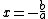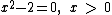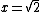# symbolic computing

(redirected from Symbolic computation)
Also found in: Wikipedia.

## symbolic computing

[sim¦bäl·ik kəm′pyüd·iŋ]
(computer science)
The development and use of symbolic systems.

## Symbolic computing

The process of manipulating numbers and variables according to the rules of mathematical logic. Variables are used to represent a real number or a set of real numbers to exact precision. Numbers can be added, subtracted, multiplied, and divided. Numerical calculations or numerical computations involve performing these operations. For example, if the symbol * is used to represent multiplication and / is used to represent division, then the equationrepresents a numerical computation whose result is 2. In mathematics, it is also possible to use symbols to represent numbers and to do computations with the symbols. For example,can also be expressed asIn this calculation or computation, the mathematical rules for subtraction and division are obeyed. The symbol x is treated as an unknown or variable that is to be solved for, and a and b as parameters representing unknown but fixed numbers.

In the 1990s, mathematical software packages became available that could manipulate symbols according to the rules of mathematics. They are also called symbolic computational packages and are programming environments, since any real number has a decimal expansion. These packages have the ability to obtain exact answers as symbols. For example, ifwere entered into a symbolic computation package, the software package would return the answerThe software package also allows the user to then ask for an approximation to 2 to any number of digits. The decimal units for 2 are determined using an algorithm that performs numerical calculation (called a numerical algorithm). Symbolic computation packages allow both symbolic and numerical computation. See Algorithm, Computer programming, Mathematical software

The capability of small computers and hand-held calculators to do extremely complicated calculations in a small amount of time led to the development of mathematical software packages that could do symbolic manipulation. These symbolic manipulation packages (also called computer algebra systems or symbolic computation systems) can do algebra, trigonometry, number theory, calculus, ordinary and partial differential equations, matrix algebra, and other areas of mathematics by manipulating the symbols according to the rules of mathematics much faster than armies of mathematicians. The answers are given exactly in symbolic form and can then be approximated numerically using routines in the package.

The advent of visualization on the computer has made it possible for these packages to plot data or functions in many forms. The functions are entered symbolically, and the software evaluates them numerically so that numbers can be plotted. The graphs generated by the plot routines can be labeled, rotated, or animated, among many other possibilities.

Hand-held calculators can perform symbolic manipulation, graph data or functions, and be programmed to carry out a sequence of operations. Mathematical software packages have been developed that can perform symbolic manipulation, graph data or functions, and be programmed using routines in the package. These packages run on most commonly used operating systems. They can also be called by computer programs to perform manipulations in the program. There are packages that do statistics, computational finance, computational physics, and computational chemistry. See Operating system, Programming languages

Mentioned in ?
References in periodicals archive ?
A double-digit Lehmer--Euclid algorithm for finding the GCD of long integers", Journal of Symbolic Computation, 19 (1995), pp.
Research Institute for Symbolic Computation, Johannes Kepler University, Altenberger Strafie 69, A-4040 Linz, Austria
The combination of Modelica with the symbolic computation power of MapleSim enables a level of understanding, power, and extensibility that is not possible with other tools.
Next, we will analyse (7) through expanding g, h, and f with the parameter [epsilon], obtain the soliton solutions by symbolic computation, and analyse the propagation characters based on numerical simulation.
Maplesoft's core technologies include the world's most advanced symbolic computation engine and revolutionary physical modeling techniques.
Aslan: Symbolic computation and exact and explicit solutions of some nonlinear evolution equation in mathematical physics, Commun.
Maplesoft, a Canadian-based maker of symbolic computation and mathematical software for scientists and engineers, is younger than these veteran companies, but not by a lot.
Hemmecke, Effective lattice points counting in rational convex polytopes, Journal of symbolic computation, 53(2003), No.
Here we illuminate the use of true mathematical software for symbolic computation to solve problems in chemical equilibrium; we illustrate the principal concepts and calculations at a level of general chemistry.
It accesses Maple's symbolic computation technology to efficiently handle all of the complex mathematics involved in the development of engineering models, including multi-domain systems, multibody systems, plant modeling, and control design.
Progress in automated computing tools and symbolic computation.

Site: Follow: Share:
Open / Close